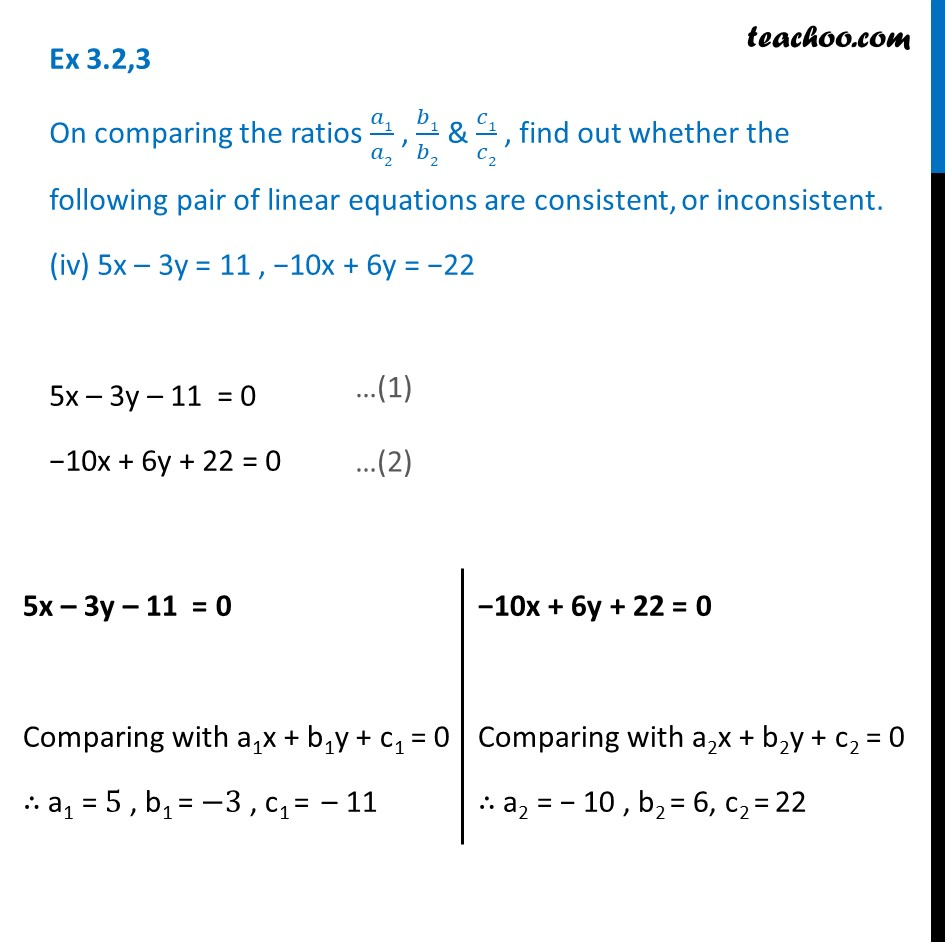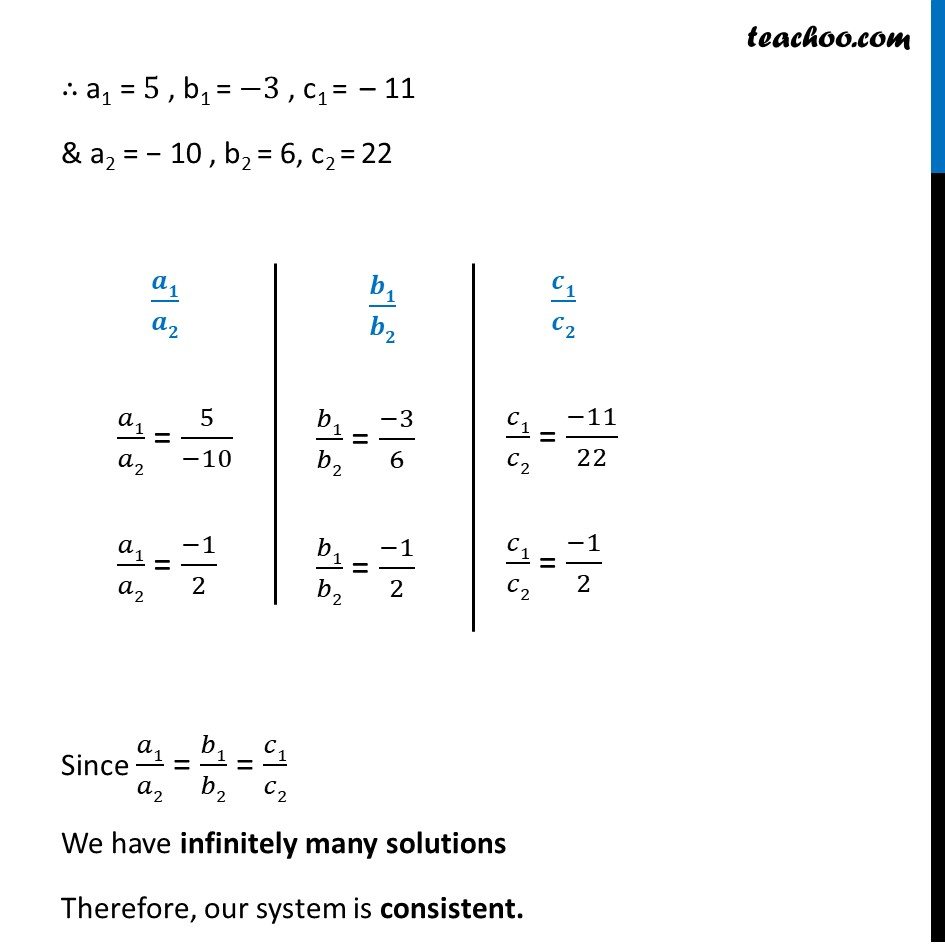1. Chapter 3 Class 10 Pair of Linear Equations in Two Variables (Term 1)
2. Serial order wise
3. Ex 3.2

Transcript

Ex 3.2,3 On comparing the ratios 𝑎1/𝑎2 , 𝑏1/𝑏2 & 𝑐1/𝑐2 , find out whether the following pair of linear equations are consistent, or inconsistent. (iv) 5x – 3y = 11 , −10x + 6y = −22 5x – 3y – 11 = 0 −10x + 6y + 22 = 0 5x – 3y – 11 = 0 Comparing with a1x + b1y + c1 = 0 ∴ a1 = 5 , b1 = −3 , c1 = – 11 −10x + 6y + 22 = 0 Comparing with a2x + b2y + c2 = 0 ∴ a2 = − 10 , b2 = 6, c2 = 22 ∴ a1 = 5 , b1 = −3 , c1 = – 11 & a2 = − 10 , b2 = 6, c2 = 22 𝒂𝟏/𝒂𝟐 𝑎1/𝑎2 = 5/(−10) 𝑎1/𝑎2 = (−1)/2 𝒃𝟏/𝒃𝟐 𝑏1/𝑏2 = (−3)/6 𝑏1/𝑏2 = (−1)/2 𝒄𝟏/𝒄𝟐 𝑐1/𝑐2 = (−11)/22 𝑐1/𝑐2 = (−1)/2 Since 𝑎1/𝑎2 = 𝑏1/𝑏2 = 𝑐1/𝑐2 We have infinitely many solutions Therefore, our system is consistent.